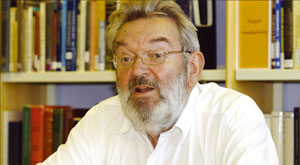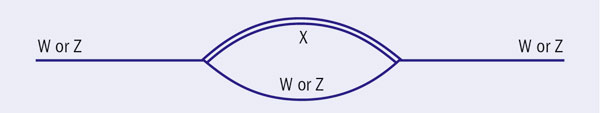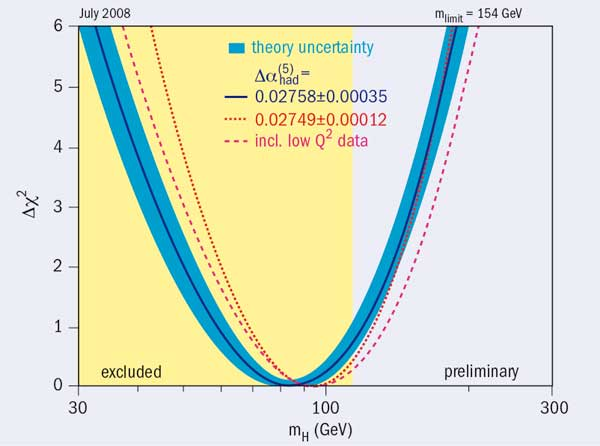# The Higgs and the LHC

20 October 2008

Understanding the Higgs sector

• Dedicated to the memory of Francisco (Paco) Ynduráin, a good friend and excellent physicist (1940–2008).

The LHC is gearing up to do real physics, and after all the astrophysical nonsense about the Big Bang and black holes we now face the cold reality of experiment. In this context, it may be useful to summarize our knowledge of the Higgs system to date, which is the purpose of this article.Martinus Veltman contemplates what the Higgs sector might reveal.

First, let me make a clear statement. Our present knowledge of the Standard Model is of course way beyond the knowledge of, say, 1959, when the PS at CERN started up. Clearly there have been many unanticipated discoveries, not to mention theoretical evolution. The Standard Model is chock full of facts crying out for explanation, such as the existence of three generations of quark–lepton families, or the many unexplained parameters of the model, such as the particle masses. That latter problem has, in today’s Standard Model, shifted to the many different particle–Higgs couplings, and is still not totally understood. Consider this: between the neutrino masses (10–3 eV) and the top quark mass (1.75 × 1011 eV) there is a difference of 14 unexplained orders of magnitude. Why? How? We can say nothing meaningful about these things and we have no idea if the LHC will illuminate the problem; at the very least, realizing all this, we should not have the arrogance to think that we know what is going to happen.

That being said, let’s see what we know. Our knowledge of the Higgs sector derives from the measurement of radiative corrections (plus the lower limit on the Higgs mass from direct experimentation at LEP), and the only quantities that depend on the details of the Higgs sector are radiative corrections to the masses of the vector bosons, including the photon. The masslessness of the photon is not automatic within the Standard Model, which provides a serious constraint.

Thus the measured radiative corrections are those affecting the W and Z masses, which come about – theoretically – through self-energy diagrams such as illustrated in figure 1. We really do not know what the X-line in the figure represents. It could be the propagator of one or more particles of distinct mass, or even some smeared-out mass (if the Higgs is heavy and strongly interacting), or of some continuous distribution. These various possibilities have been scrutinized for quite some time, but no definite view has emerged. Even so, it is useful to take a specific model, namely the simplest Higgs sector with one physical Higgs.Radiative corrections to the W and Z masses arise through self-energy diagrams like this.

In the first instance, in a renormalizable theory, masses are free parameters – to be renormalized and taken from experiment – and therefore radiative corrections are invisible. Nonetheless there are two facts that allow us to come to some conclusions.

The first fact is that the photon mass is zero. Such a mass is not subject to renormalization and we may thus ask under what circumstances the Higgs sector produces a photon mass of zero. It happens that the simplest Higgs model (with just one physical Higgs) produces a massless photon, while adding degrees of freedom to the Higgs sector in general destroys this prediction. To get a zero photon mass in more complicated Higgs systems requires tuning of parameters, in other words, the prediction of zero photon mass is lost. This, in my opinion, is a strong argument against more complicated Higgs systems. To abandon a prediction that agrees with experiment is not something one should do lightly. However, this is not without some nasty consequences.

Here I must mention the strong CP violation contained in the strong interactions in QCD. This effect, which indeed is not observed, is commonly explained away (in the Peccei–Quinn approach) by using two Higgs systems. While it is easy within such a model to tune the photon mass to zero, it is nonetheless a fact that the prediction of zero mass is lost. On top of that, in these models there is normally a particle of very small mass (the axion) of which there is no evidence experimentally. This is a worrying problem, for which there is no generally accepted solution, although there are some attempts at resolving it.The “Δχ2” curve derived from high-Q2 precision electroweak measurements, performed at LEP and by SLD, CDF, and D0, as a function of the Higgs-boson mass, assuming the Standard Model to be the correct theory of nature. The yellow area is excuded by the non-production of the Higgs. Image credit: LEP Electroweak Working Group.

In addition, there is supersymmetry. In supersymmetry one inevitably has more than one Higgs system, so a priori that ruins the prediction of zero photon mass of the simplest Higgs model. The simplest supersymmetric model accidentally escapes this problem and predicts a zero photon mass; however there are other difficulties with this model.

The second fact concerns the vector-boson masses. The simplest Higgs model predicts a certain ratio between the W and Z masses, which is not subject to renormalization. The associated parameter is the r parameter and, assuming that the only things that change the ratio arise from radiative corrections, one obtains a prediction for the Higgs mass (assuming the simplest model). This is the source of the predictions on the limits on the Higgs mass that are commonly quoted (figure 2). It should be pointed out that the corrections to the mass ratio also contain a prediction for the top quark mass that agrees very well with the observed value. So, indeed, we must assume that the Higgs sector is such that the prediction for the mass ratio of the W and Z bosons is that given by the simplest Higgs sector. This puts severe limits on theoretical models for the Higgs sector.

It is clear that our knowledge of the Higgs sector is scanty, and in particular a Higgs system with a very heavy Higgs is quite possible. The latter would probably produce a wide resonance for the X in figure 1, and it would be hard to make precise statements on the decays of such a resonance. Well, let us hope that the LHC clarifies the matter.